SLPS546A July   2015  – March 2017

PRODUCTION DATA.

1. 1Features
2. 2Applications
3. 3Description
4. 4Revision History
5. 5Specifications
6. 6Application and Implementation
1. 6.1 Application Information
2. 6.2 Typical Application
3. 6.3 System Example
7. 7Layout
1. 7.1 Layout Guidelines
2. 7.2 Layout Example
3. 7.3 Thermal Considerations
8. 8Device and Documentation Support
9. 9Mechanical, Packaging, and Orderable Information

• DPB|8

## 5 Specifications

### 5.1 Absolute Maximum Ratings

TA = 25°C (unless otherwise noted) (see (1))
MIN MAX UNIT
Voltage VIN to PGND 30 V
VSW to PGND 30
VSW to PGND (10 ns) 32
TG to TGR –0.3 10
BG to PGND –0.3 10
IDM Pulsed current rating 60 A
PD Power dissipation 6 W
EAS Avalanche energy Sync FET, ID = 31 A, L = 0.1 mH 48 mJ
Control FET, ID = 31 A, L = 0.1 mH 48
TJ Operating junction temperature –55 150 °C
Tstg Storage temperature –55 150 °C
Stresses beyond those listed under Absolute Maximum Ratings may cause permanent damage to the device. These are stress ratings only, and functional operation of the device at these or any other conditions beyond those indicated in the Recommended Operating Conditions is not implied. Exposure to absolute-maximum-rated conditions for extended periods may affect device reliability.

### 5.2 Recommended Operating Conditions

TA = 25°C (unless otherwise noted)
MIN MAX UNIT
VGS Gate drive voltage 3.3 8 V
VIN Input supply voltage 24 V
ƒSW Switching frequency CBST = 0.1 µF (min) 1500 kHz
Operating current 20 A
TJ Operating temperature 125 °C

### 5.3 Power Block Performance

TA = 25°C (unless otherwise noted) (see (1))
PARAMETER TEST CONDITIONS MIN TYP MAX UNIT
PLOSS Power loss(1) VIN = 12 V, VGS = 5 V, VOUT = 3.3 V,
IOUT = 12 A, ƒSW = 500 kHz,
LOUT = 1 µH, TJ = 25°C
1.6 W
IQVIN VIN quiescent current TG to TGR = 0 V BG to PGND = 0 V 10 µA
Measurement made with six 10-µF (TDK C3216X5R1C106KT or equivalent) ceramic capacitors placed across VIN to PGND pins and using a high current 5-V driver IC.

### 5.4 Thermal Information

TA = 25°C (unless otherwise stated)
THERMAL METRIC MIN TYP MAX UNIT
RθJA Junction-to-ambient thermal resistance (min Cu)(2) 130 °C/W
Junction-to-ambient thermal resistance (max Cu)(2)(1) 75
RθJC Junction-to-case thermal resistance (top of package)(2) 21 °C/W
Junction-to-case thermal resistance (PGND pin)(2) 2.1
Device mounted on FR4 material with 1-in2 (6.45-cm2) Cu.
RθJC is determined with the device mounted on a 1-in2 (6.45-cm2), 2-oz (0.071-mm) thick Cu pad on a 1.5-in × 1.5-in
(3.81-cm × 3.81-cm), 0.06-in (1.52-mm) thick FR4 board. RθJC is specified by design while RθJA is determined by the user’s board design.

### 5.5 Electrical Characteristics

TA = 25°C (unless otherwise stated)
PARAMETER TEST CONDITIONS Q1 CONTROL FET Q2 SYNC FET UNIT
MIN TYP MAX MIN TYP MAX
STATIC CHARACTERISTICS
BVDSS Drain-to-source voltage VGS = 0 V, IDS = 250 µA 30 30 V
IDSS Drain-to-source leakage current VGS = 0 V, VDS = 20 V 1 1 µA
IGSS Gate-to-source leakage current VDS = 0 V, VGS = 10 V 100 100 nA
VGS(th) Gate-to-source threshold voltage VDS = VGS, IDS = 250 µA 0.75 0.90 1.20 0.75 0.90 1.20 V
RDS(on) Drain-to-source on resistance VGS = 3.5 V, IDS = 12 A 6.3 8.3 6.3 8.3
VGS = 4.5 V, IDS = 12 A 5.6 7.0 5.6 7.0
VGS = 8 V, IDS = 12 A 4.9 6.0 4.9 6.0
gfs Transconductance VDS = 15 V, IDS = 12 A 62 62 S
DYNAMIC CHARACTERISTICS
CISS Input capacitance VGS = 0 V, VDS = 15 V,
ƒ = 1 MHz
971 1260 971 1260 pF
COSS Output capacitance 453 589 453 589 pF
CRSS Reverse transfer capacitance 16 21 16 21 pF
RG Series gate resistance 1.0 2.0 1.0 2.0 Ω
Qg Gate charge total (4.5 V) VDS = 15 V,
IDS = 12 A
6.4 8.3 6.4 8.3 nC
Qgd Gate charge gate-to-drain 1.0 1.0 nC
Qgs Gate charge gate-to-source 1.9 1.9 nC
Qg(th) Gate charge at Vth 0.9 0.9 nC
QOSS Output charge VDS = 15 V, VGS = 0 V 10.5 10.5 nC
td(on) Turnon delay time VDS = 15 V, VGS = 4.5 V,
IDS = 12 A, RG = 2 Ω
4 4 ns
tr Rise time 7 7 ns
td(off) Turnoff delay time 11 11 ns
tf Fall time 17 17 ns
DIODE CHARACTERISTICS
VSD Diode forward voltage IDS = 12 A, VGS = 0 V 0.8 1.0 0.8 1.0 V
Qrr Reverse recovery charge VDS = 15 V, IF = 12 A,
di/dt = 300 A/µs
23 23 nC
trr Reverse recovery Time 18 18 ns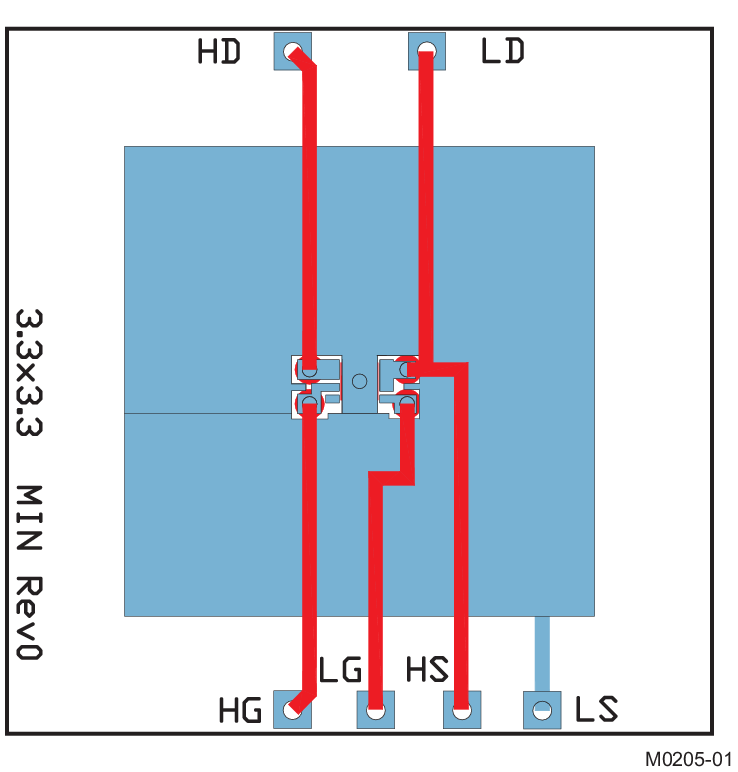Max RθJA = 75°C/W when mounted on 1 in2 (6.45 cm2) of 2-oz (0.071-mm) thick Cu.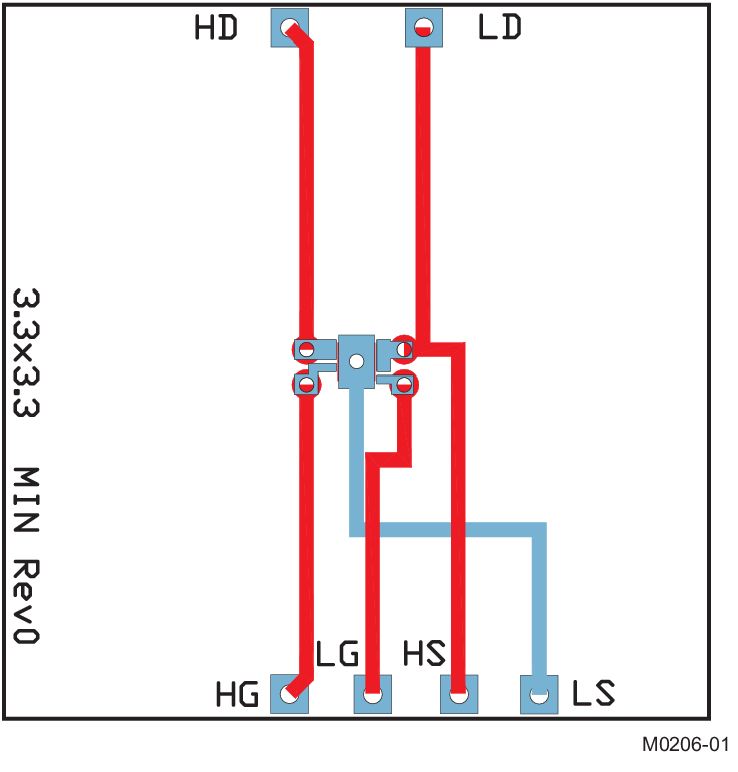Max RθJA = 130°C/W when mounted on minimum pad area of 2-oz (0.071-mm) thick Cu.

### 5.6 Typical Power Block Device Characteristics

The typical power block system characteristic curves (Figure 1 through Figure 9) are based on measurements made on a PCB design with dimensions of 4 in (W) × 3.5 in (L) × 0.062 in (H) and 6 copper layers of 1-oz copper thickness. See Application and Implementation for detailed explanation. Conditions for Figure 1 through Figure 5 are given by the following; VIN = 12 V, VGS = 5 V, VOUT = 3.3 V, ƒSW = 500 kHz, LOUT = 1 µH. TA = 125°C, unless stated otherwise.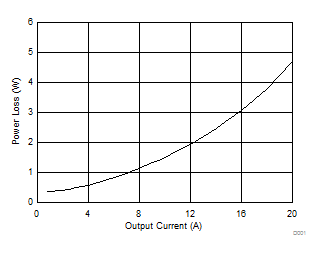Figure 1. Power Loss vs Output Current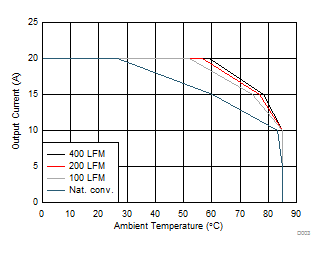Figure 3. Safe Operating Area – PCB Horizontal Mount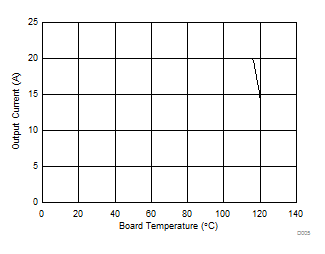Figure 5. Typical Safe Operating AreaFigure 2. Power Loss vs Temperature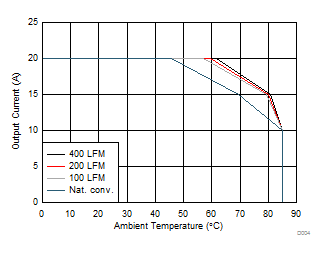Figure 4. Safe Operating Area – PCB Vertical Mount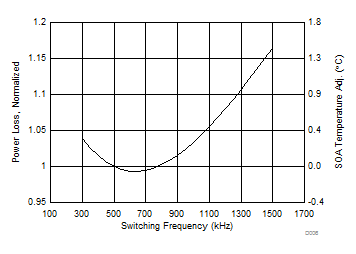VIN = 12 V VGS = 5 V VOUT = 3.3 V IOUT = 15 A LOUT = 1.0 µH
Figure 6. Normalized Power Loss vs Switching Frequency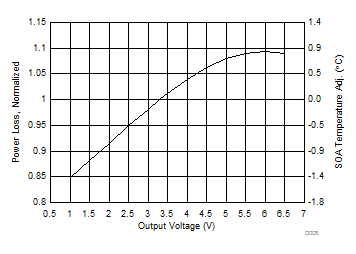VIN = 12 V VGS = 5 V IOUT = 15 A ƒSW = 500 kHz LOUT = 1.0 µH
Figure 8. Normalized Power Loss vs Output Voltage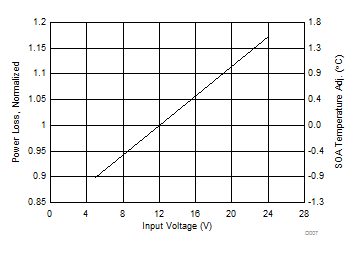ƒSW = 500 kHz VGS = 5 V VOUT = 3.3 V IOUT = 15 A LOUT = 1.0 µH
Figure 7. Normalized Power Loss vs Input Voltage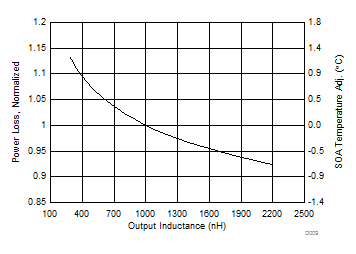VIN = 12 V VGS = 5 V IOUT = 15 A ƒSW = 500 kHz VOUT = 3.3 V
Figure 9. Normalized Power Loss vs Output Inductance

### 5.7 Typical Power Block MOSFET Characteristics

TA = 25°C, unless stated otherwise.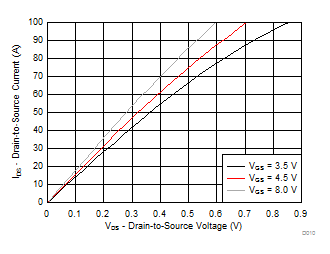Figure 10. MOSFET Saturation Characteristics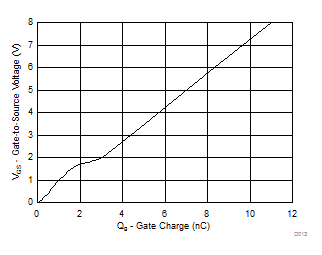ID = 12 A VDS = 15 V
Figure 12. MOSFET Gate Charge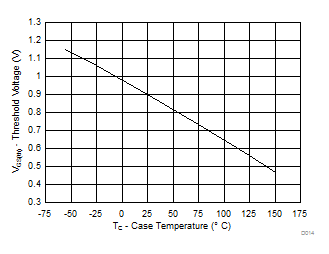ID = 250 µA
Figure 14. MOSFET VGS(th)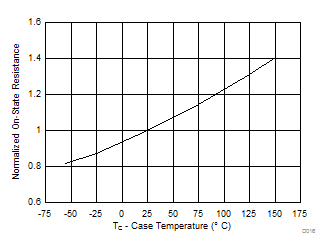ID = 12 A
Figure 16. MOSFET Normalized RDS(on)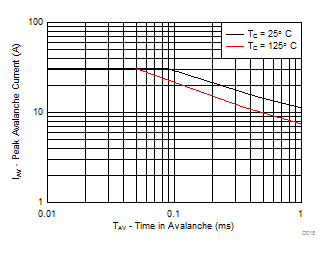Figure 18. MOSFET Unclamped Inductive Switching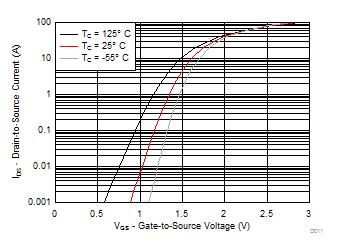VDS = 5 V
Figure 11. MOSFET Transfer Characteristics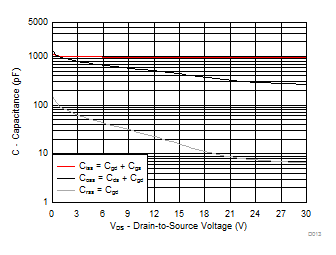Figure 13. MOSFET Capacitance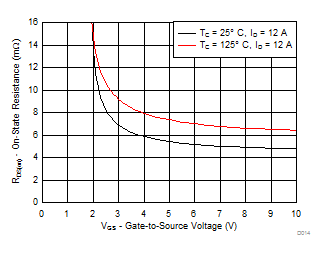Figure 15. MOSFET RDS(on) vs VGS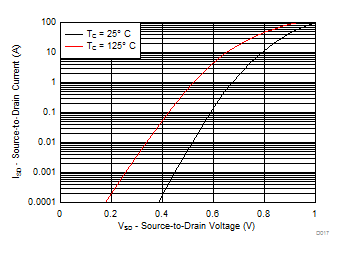Figure 17. MOSFET Body Diode